## 4.2.1 2D Rigid Bodies: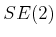Section 3.2.2 expressed how to transform a rigid body in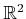by a homogeneous transformation matrix,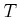, given by (3.35). The task in this chapter is to characterize the set of all possible rigid-body transformations. Which manifold will this be? Here is the answer and brief explanation. Since any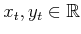can be selected for translation, this alone yields a manifold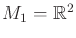. Independently, any rotation,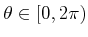, can be applied. Sinceyields the same rotation as 0, they can be identified, which makes the set of 2D rotations into a manifold,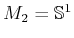. To obtain the manifold that corresponds to all rigid-body motions, simply take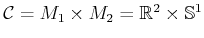. The answer to the question is that the C-space is a kind of cylinder.

Now we give a more detailed technical argument. The main purpose is that such a simple, intuitive argument will not work for the 3D case. Our approach is to introduce some of the technical machinery here for the 2D case, which is easier to understand, and then extend it to the 3D case in Section 4.2.2.

Subsections
Steven M LaValle 2012-04-20